# JAC Class 9 Maths Solutions Chapter 10 Circles Ex 10.4

Jharkhand Board JAC Class 9 Maths Solutions Chapter 10 Circles Ex 10.4 Textbook Exercise Questions and Answers.

## JAC Board Class 9th Maths Solutions Chapter 10 Circles Ex 10.4

Page-179

Question 1.
Two circles of radii 5 cm and 3 cm intersect at two points and the distance between their centres is 4 cm. Find the length of the common chord.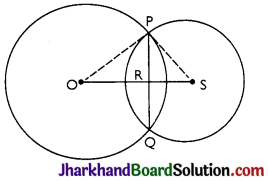OP = 5 cm, PS = 3 cm and OS = 4 cm.
We know that if two circles intersect at two points then their centres lie on the perpendicular bisector of the common chord.
So, OS is perpendicular bisector of PQ.
⇒ PQ = 2PR and ∠PRO = ∠PRS = 90°
Let RS be x ⇒ OR = 4 – x
In ∆POR,
OP2 = OR2 + PR2
⇒ 52 = (4 – x)2 + PR2
⇒ 25 = 16 + x2 – 8x + PR2
⇒ PR2 = 9 – x2 + 8x …(i)

In ∆PRS,
PS2 – PR2 + RS2
⇒ 32 = PR2 + x2
⇒ PR2 = 9 – x2 …(ii)
Equating (i) and (ii),
9 – x2 + 8x = 9 – x2
⇒ 8x = 0
⇒ x = 0
Putting the value of x in (i), we get
PR2 – 9 – 02
⇒ PR = 3 cm
Length of the Chord PQ = 2PR = 2 × 3 = 6 cmQuestion 2.
If two equal chords of a circle intersect within the circle, prove that the segments of one chord are equal to corresponding segments of the other chord.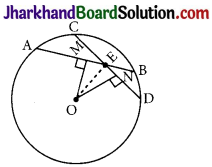Given: AB and CD are chords intersecting at E.
AB = CD
To prove: AE = DE and CE = BE
Construction: OM ⊥ AB and ON ⊥ CD. OE is joined.
Proof: OM bisects AB (OM ⊥ AB)
⇒ AM = MB = $$\frac{1}{2}$$ AB
ON bisects Cp (ON ⊥ CD)
⇒ DN = CN = $$\frac{1}{2}$$ CD
As AB = CD
thus, AM = ND …….(i)
and MB = CN ……..(ii)

In ∆OME and ∆ONE,
∠OME = ∠ONE = 90° (Perpendiculars)
OE = OE (Common)
OM = ON (AB = CD and thus equidistant from the centre)
∆OME ≅ ∆ONE by RHS congruence criterion.
ME = EN by CPCT …(iii)
Adding (i) and (ii), we get
AM + ME = ND + EN
⇒ AE = ED
Subtracting (iii) from (ii), we get
MB – ME = CN – EN
⇒ EB = CE

Question 3.
If two equal chords of a circle intersect within the circle, prove that the line joining the point of intersection to the centre makes equal angles with the chords.
Given: AB and CD are chords intersecting at E.
AB = CD, PQ is the diameter.
To prove: ∠BEQ = ∠CEQ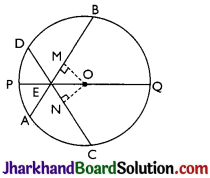Construction: OM ⊥ AB and ON ⊥ CD. OE is joined.
In ∆OEM and ∆OEN,
OM = ON (AB = CD and Equal chords are equidistant from the centre)
OE = OE (Common)
∠OME = ∠ONE = 90° (Perpendiculars)
∆OEM = ∆OEN (by RHS congruence criterion)
Thus, ∠OME = ∠ONE (by CPCT)
⇒ ∠BEQ = ∠CEQQuestion 4.
If a line intersects two concentric circles (circles with the same centre) with centre O at A, B, C and D, prove that AB = CD (see Fig).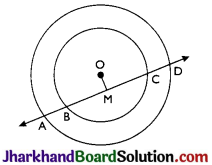OM ⊥ AD is drawn from O.
So, OM bisects AD (perpendicular from centre to the chord bisects the chord)
⇒ AM = MD …(i)
Also, OM bisects BC as OM ⊥ BC.
⇒ BM = MC …(ii)
Subtracting (ii) from (i), we get
AM – BM = MD – MC
⇒ AB = CD

Question 5.
Three girls Reshma, Salma and Mandip are playing a game by standing on a circle of radius 5 m drawn in a park. Reshma throws a ball to Salma, Salma to Mandip, Mandip to Reshma. If the distance between Reshma and Salma and between Salma and Mandip is 6 m e ach, what is the distance between Reshma and Mandip?
Let A, B and C represent the positions of Reshma, Salma and Mandip respectively.
AB = 6 m and BC = 6 m Radius OA = 5 m
BM ⊥ AC is drawn.
ABC is an isosceles triangle as AB = BC, M is mid-point of AC. BM is perpendicular bisector of AC and thus it passes through the centre of the circle.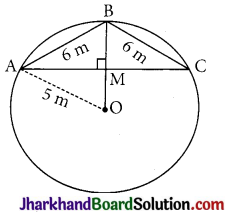Let, AM = y and OM = x then BM = (5 – x).
Applying Pythagoras theorem in ∆OAM,
OA2 = OM2 + AM2
⇒ 52 = x2 + y2 …(i)
Applying Pythagoras theorem in ∆AMB,
AB2= BM2 +AM2
⇒ 62 = (5-x)2 + y2 …(ii)
Subtracting (i) from (ii), we get
36 – 25 = (5 – x)2 – x2
⇒ 11 = 25 – 10x
⇒ 10x = 14
⇒ x = $$\frac{7}{5}$$

Substituting the value of x in (i), we get
y2 + $$\frac{49}{25}$$ =25
⇒ y2 = 25 – $$\frac{49}{25}$$
⇒ y2 = $$\frac{625-49}{25}$$
⇒ y2 = $$\frac{576}{25}$$
⇒ y = $$\frac{24}{5}$$

Thus,
AC = 2 × AM = 2 × y
= 2 × $$\frac{24}{5}$$ m = $$\frac{48}{5}$$ m = 9.6 m
Distance between Reshma and Mandip is 9.6 m.Question 6.
A circular park of radius 20 m is situated in a colony. Three boys Ankur, Syed and David are sitting at equal distance on its boundary each having a toy telephone in his hands to talk each other. Find the length of the string of each phone.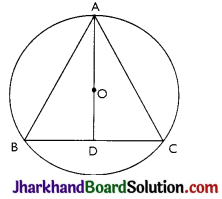Let A, B and C represent the positions of Ankur, Syed and David respectively. All three boys at equal distances thus, ABC is an equilateral triangle.
Now, AD is median of ∆ABC and it passes through the centre O.
Also, O is the centroid of the ∆ABC.
OA is the radius of the triangle.
OA = $$\frac{2}{3}$$ AD
Let the side of the triangle be a metres
then BD = $$\frac{a}{2}$$ m.
Applying Pythagoras theorem in ∆ABD,
⇒ AD2 = a2 – ($$\frac{a}{2}$$)2
⇒ AD2 = $$\frac{3 a^2}{4}$$
⇒ AD = $$\frac{\sqrt{3} a}{2}$$
OA = $$\frac{2}{3}$$ AD
⇒ 20m = $$\frac{2}{3}$$ × $$\frac{\sqrt{3} a}{2}$$
⇒ a = $$20 \sqrt{3}$$m
∴ Length of the string is $$20 \sqrt{3}$$m